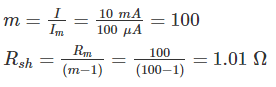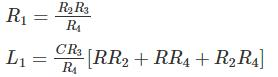Courses

# Measurements And Instruments 3 MCQ

## 20 Questions MCQ Test Mock Test Series for SSC JE Electrical Engineering (Hindi) | Measurements And Instruments 3 MCQ

Description
This mock test of Measurements And Instruments 3 MCQ for Electrical Engineering (EE) helps you for every Electrical Engineering (EE) entrance exam. This contains 20 Multiple Choice Questions for Electrical Engineering (EE) Measurements And Instruments 3 MCQ (mcq) to study with solutions a complete question bank. The solved questions answers in this Measurements And Instruments 3 MCQ quiz give you a good mix of easy questions and tough questions. Electrical Engineering (EE) students definitely take this Measurements And Instruments 3 MCQ exercise for a better result in the exam. You can find other Measurements And Instruments 3 MCQ extra questions, long questions & short questions for Electrical Engineering (EE) on EduRev as well by searching above.
QUESTION: 1

### Which of the following is not an Integrating instrument?

Solution:

Integrating instruments totalize events over a specified period of time. The summation, which they give is the product of time and electrical quantity. Ampere hour and watt hour (energy) meters are examples of this category.

Voltmeter is not an integrating type of instrument

Indicating instruments are those instruments which indicate the magnitude of a quantity being measured. They generally make use of a dial and a pointer for this purpose. Ordinary voltmeters, ammeters and wattmeters belong to this category

QUESTION: 2

### Which of the following constitutes the most important specification of an instrument?

Solution:

Range is the most important specification of an instrument. It indicates the range of values which the instrument can measure. We will discuss the importance of range with an example.

Suppose we need to measure 10 mA quantity. So we need an ammeter with the full scale range of at least 10 mA. Hence range is useful when selecting the meter to measure the quantity

QUESTION: 3

### Calculate the limiting error of an ammeter of range 25 A given also that it has a guaranteed accuracy of 1% of full-scale reading. The current measured by the ammeter is 5 A.

Solution:

Given that, GAE = 1% of full scale reading

Full scale reading = 25 A

Error value = 0.01 × 25 = 0.25 AQUESTION: 4

Solution:

When a voltmeter having an internal resistance of Rm is connected in parallel with load resistance RL of circuit under test, the circuit conditions will be altered. The effective resistance will be the parallel combination of RL and Rm. The voltmeter indicates the voltage across this effective resistance, where the indicated voltage will always be less than true voltage. This is known as loading effect.

Hence the instrument must possess high input impedance to reduce loading effect.

QUESTION: 5

The most suitable material for spring in majority of the measuring instruments, except in low resistance instruments is:

Solution:

The essential requirements for instrument for instrument springs are:

1) They should be non-magnetic

2) They should be proof from mechanical fatigue

3) Where springs are used to lead current into moving system they should have a small resistance, their cross-sectional area must be sufficient to carry the current without temperature rise affecting their constant. They should also have a low resistance temperature coefficient.

For most applications phosphor bronze has been found to be the most suitable material except in instruments low in resistance (like multi-voltmeters).

QUESTION: 6

In a gravity controlled instrument, the deflection is proportional to:

Solution:

In gravity control, a small weight is placed on an arm attached to the moving system. The position of this weight is adjustable. This weight produces a controlling torque due to gravity.

This controlling torque is given by,

Tc = Kgsinθ

T∝ I

At equilibrium position, TC = TD

⇒ I ∝ sinθ

QUESTION: 7

Indicating instruments should be

Solution:

The damping torque should be of such a magnitude that the pointer quickly comes to its final steady state position without overshooting.

If the instrument is underdamped, the moving system will oscillate about the final steady position with a decreasing amplitude and will take some time before it comes to rest.

When the system moves rapidly but smoothly to its final steady position, the instrument is said to be critically damped.

If the damping torque is more than what is required for critical damping, the instrument is said to be overdamped. In an overdamped instrument, the moving system moves slowly to its final steady state position in a lethargic fashion.

QUESTION: 8

Which instruments have uniform scale-

Solution:

In spring control instrument, the controlling torque is given by,

Tc = Kcθ

T∝ I

At equilibrium position, TC = TD

⇒ I ∝ θ

Hence in spring control, the scale is uniform

In gravity control, a small weight is placed on an arm attached to the moving system. The position of this weight is adjustable. This weight produces a controlling torque due to gravity.

This controlling torque is given by,

Tc = Kgsinθ

Td ∝ I

At equilibrium position, TC = TD

⇒ I ∝ sinθ

Hence in gravity control, the scale is non uniform

QUESTION: 9

A meter movement with current sensitivity 100 μA and internal resistance 100 Ω is required to measure current of 10mA. What will the shunt resistance be equal to?

Solution:

Given that,

R= 100 Ω

I= 10 mA

I = 100 μAQUESTION: 10

Two wattmeter method can be used to measure the total power delivered to:

Solution:

Two wattmeter method can be used to measure the total power delivered to both start as well as delta connected loads.

QUESTION: 11

In an electrodynamometer type wattmeter:

Solution:

It has two fixed coils and one moving coil. The field is produced by these fixed coils which is required for the operation of the instrument. A uniform field is obtained at the centre of the coil due to division of coil in two sections. It is used as current coil.

The moving coil is mounted on an aluminium spindle. It used as pressure coil or voltage coil.

QUESTION: 12

Single or One Wattmeter method can only be used for

Solution:

Single or one wattmeter method is used for only balanced three phase loads. It is best method for the measurement of 3 phase reactive power compared to 2 wattmeter method since error can be minimized.

QUESTION: 13

The loss of charge method can be used to measure which of the following?

Solution:

The loss of charge method is used to measure high values of resistance. This method is best suitable for measurement of insulation resistance in case of cable.

QUESTION: 14

Copper shading is provided in energy meter to

Solution:

The copper shading bands are also called the power factor compensators. These rings are provided on the central limb of the shunt magnet to make the phase angle displacement between magnetic field set up by shunt magnet and supply voltage is approximately 90 degrees.

QUESTION: 15

The e.m.f. developed by a thermocouple depends upon

Solution:

A thermocouple is a device used extensively for measuring temperature. It comprises of two dissimilar metals, A and B. These are joined together to form two junctions, p and q, which are maintained at the temperatures T1 and T2 respectively. Since the two junctions are maintained at different temperatures the Peltier emf is generated within the circuit and it is the function of the temperatures of two junctions.

If the temperature of both the junctions is same, equal and opposite emf will be generated at both junctions and the net current flowing through the junction is zero.

If the junctions are maintained at different temperatures, the emf’s will not become zero and there will be a net current flowing through the circuit.

The total emf flowing through this circuit depends on the metals used within the circuit as well as the temperature of the two junctions.

QUESTION: 16

The technique of using stair case ramp in DVM (Digital Volt Meter) is called

Solution:

The ramp type DVM uses a linear ramp or staircase ramp technique. The staircase ramp technique is a simpler version of linear ramp technique. It is called as null balancing technique.

QUESTION: 17

Sensitivity of LVDT is mainly due to

Solution:

LVDT (Linear Variable Differential Transformer) is the most widely used inductive transducer that coverts the linear motion into the electrical signal. Sensitivity of LVDT is mainly due to exact cancellation of secondary voltages

QUESTION: 18

Horizontal deflection plates of CRO are placed generally:

Solution:

The horizontal and vertical plates are placed between the electron gun and the screen, thus it can detect the beam according to the input signal. Generally these horizontal deflection plates are placed vertically.

QUESTION: 19

Which expression depicts the sensitivity (S) of a Wheatstone bridge?

(Where: D is Deflection and I is current)

Solution:

Sensitivity (S) of a Wheatstone bridge is expressed as,

S = D/I

Where: D is Deflection and I is current

The Wheatstone bridge is more sensitive when all their resistances are equal, or their ratio is unity. Their sensitivity decreases when their ratio is less than unity. The reduction in sensitivity reduces the accuracy of the bridge.

QUESTION: 20

The unknown inductance is measured in terms of _________ in the case of Anderson bridge

Solution:

In Anderson bridge, the unknown inductance and resistance values are,The unknown inductance is measured in terms of known resistance and capacitance.